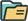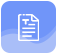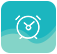# 2021年自考《高等数学二》备考习题及答案1

### 来源：成人学历报考中心发布日期：2022-04-22

1.下列命题正确的是( )

A函数f(x)的导数不存在的点，一定不是f(x)的极值点

B若x0为函数f(x)的驻点，则x0必为f(x)的极值点

C若函数f(x)在点x0处有极值，且f'(x0)存在，则必有f'(x0)=0

D若函数f(x)在点x0处连续，则f'(x0)一定存在

正确答案：C

2.设函数f(x)在x=1处可导，且f'(1)=0，若f'(1)>0，则f(1)是( )

A极大值

B极小值

C不是极值

D是拐点

正确答案：B

3.设函数y=sin(x2-1)，则dy等于( ).

A cos(x2-1)dx

B-cos(x2-1)dx

C 2xcos(x2-1)dx

D-2xcos(x2-1)dx

正确答案：C

4.设函数y=e2x+5，则y'=( ).

A e2x

B 2e2x

C 2e2x+5

D 2ex+5

正确答案：B

5.函数y=(x)在点x=0处的二阶导数存在，且'(0)=0，'(0)>0，则下列结论正确的是( ).

A x=0不是函数(x)的驻点

B x=0不是函数(x)的极值点

C x=0是函数(x)的极小值点

D x=0是函数(x)的极大值点

正确答案：C

6.设函数(x)在x=0处连续，当x<0时，'(x)<0;当x>0时，，(x)>0.则( ).

A(0)是极小值

B(0)是极大值

C(0)不是极值

D(0)既是极大值又是极小值

正确答案：A

7.f(x)=|x-2|在点x=2的导数为( )

A 1

B 0

C-1

D不存在

正确答案：D

8.若f'(x)<0(a0，则在(a，b)内必有( )

A f(x)>0

B f(x)<0

C f(x)=0

D f(x)符号不定

正确答案：A

9.设函数(x)=exlnx，则'(1)=( ).

A 0

B 1

C e

D 2e

正确答案：C

10.d(sin2x)=( ).

A 2cos2xdx

B cos2xdx

C-2cos2xdx

D-cos2xdx

正确答案：A

11.设函数(x)=cos2x，则'(x)=( ).

A 2sin2x

B-2sin2x

C sin2x

D-sin2x

正确答案：B

## 声明：

（一）由于考试政策等各方面情况的不断调整与变化，本网站所提供的考试信息仅供参考，请以权威部门公布的正式信息为准。

（二）本网站在文章内容来源出处标注为其他平台的稿件均为转载稿，免费转载出于非商业性学习目的，版权归原作者所有。如您对内容、版权等问题存在异议请与本站联系，我们会及时进行处理解决。题库资料
•真题精炼
依据历年真题，快速梳理
知识点，检验学习效果
点击查看
•模拟试题
依据考试大纲高质量
模拟试卷
点击查看
•教材大纲
全力冲刺，紧抓考前机会
全力冲考
点击查看